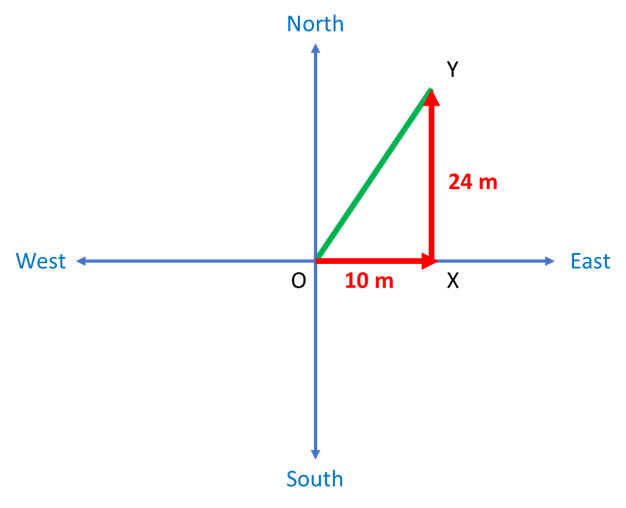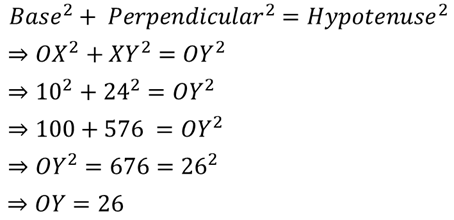Courses
Courses for Kids
Free study material
Offline Centres
MoreLast updated date: 01st Dec 2023
Total views: 385.2k
Views today: 10.85k

# Aniket goes 10 m due east and then 24 m due north. Find his distance from the starting point.A) 10 mB) 16 mC) 26 mD) 30 mVerified
385.2k+ views
Hint:

In such questions, "distance from the starting point" refers to the shortest distance between the start point and the end point. Most of these questions involve the creation of right-angled triangles and then applying the Pythagoras Theorem. It is advisable to draw a diagram and then solve such problems.Try to solve this yourself before looking at the complete solution.

Let us assume Aniket starts at point 'O'. First he moves east 10 m to 'X'. Next he moves north 24 m to 'Y'. As can be seen from the diagram, his distance from the starting point (meaning shortest distance) is OY. Therefore, finding out the length of OY will give us the answer.

I. We know that the directions East and North are perpendicular to each other. Therefore, we can say that the triangle OXY is a right-angled triangle at X.

II. As OXY is a right-angled triangle, we can apply Pythagoras Theorem here. Pythagoras Theorem states that:Hence, Aniket is 26 m from his starting point. (Option (C))

Note:

Such questions can be solved quickly if you can remember the Pythagorean Triplets. Below are a few:
(3, 4, 5)

(5, 12, 13)

(7, 24, 25)

This question used the (5, 12, 13) triplet... just multiply the complete set by 2 and you get another triplet
(5, 12, 13) * 2 gives (10, 24, 26)

As soon as you saw the sides are 10 and 24, you could tell the hypotenuse length was 26!